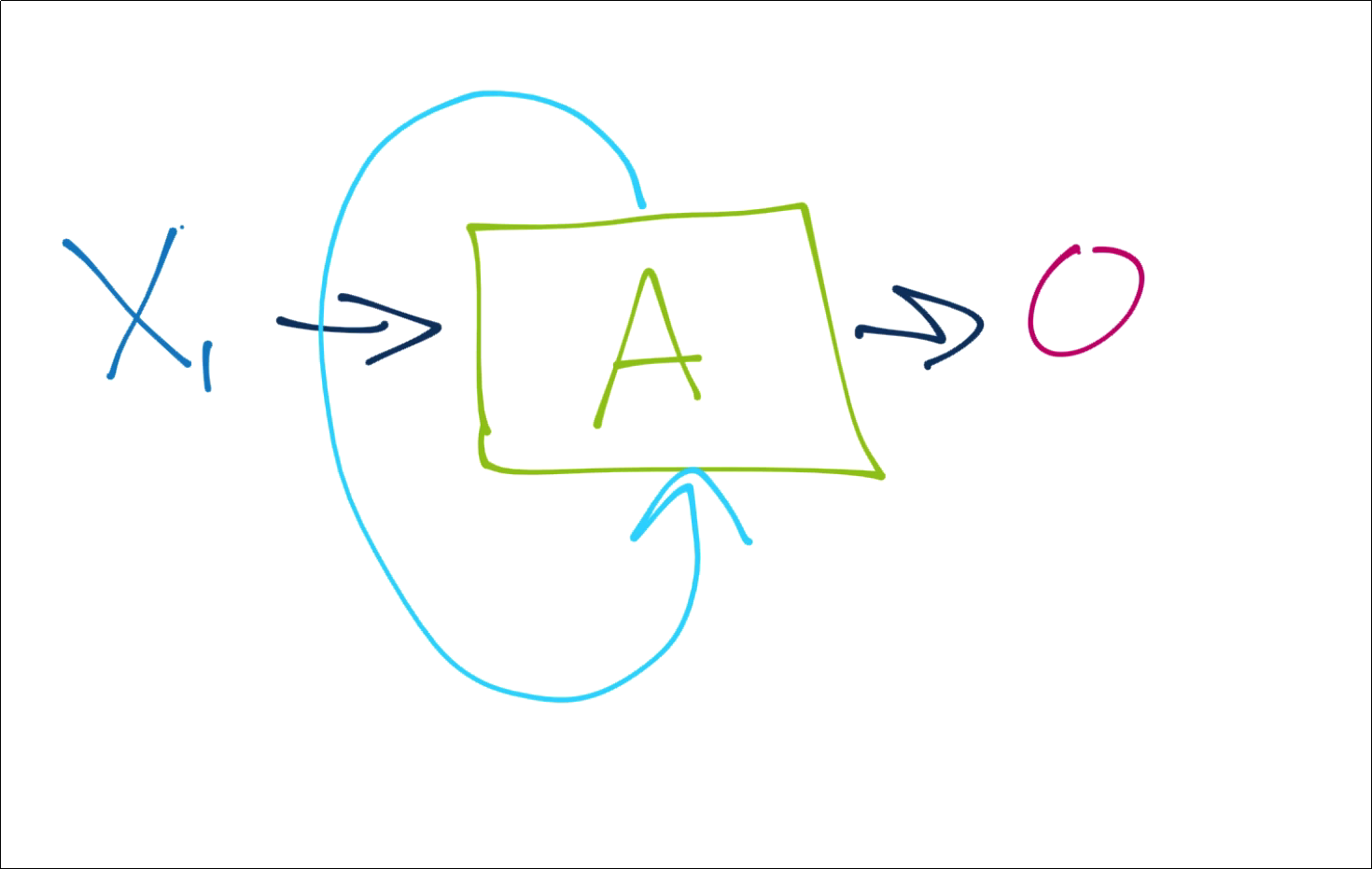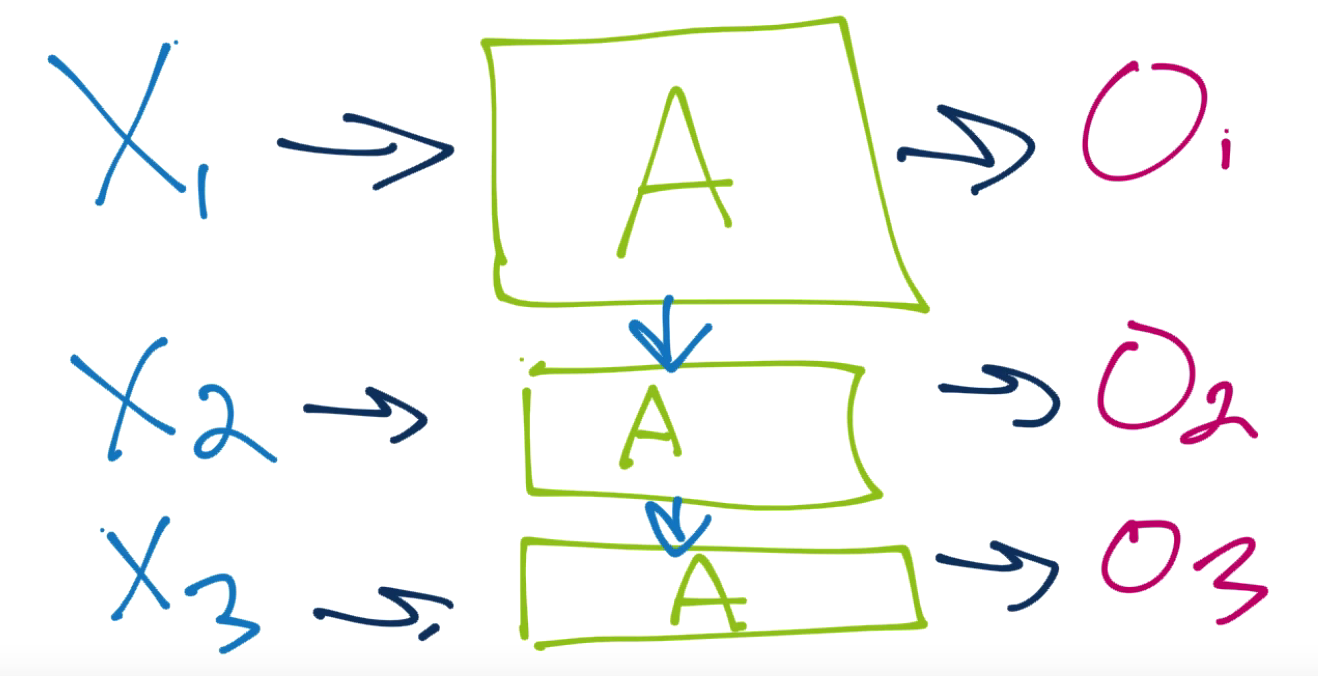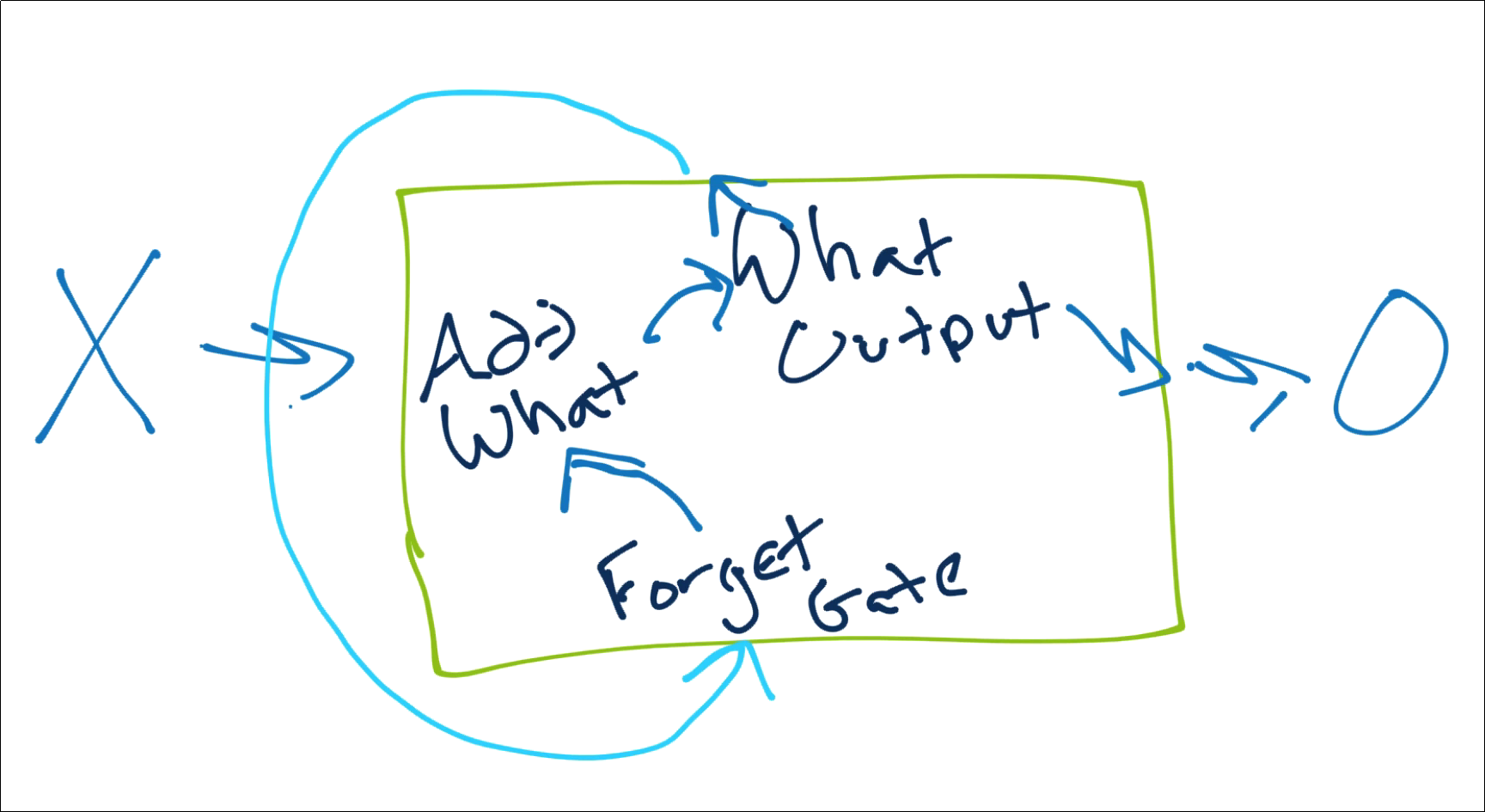## Recurrent Neural Network (RNN) basics and the Long Short Term Memory (LSTM) cell

Welcome to part ten of the Deep Learning with Neural Networks and TensorFlow tutorials. In this tutorial, we're going to cover the Recurrent Neural Network's theory, and, in the next, write our own RNN in Python with TensorFlow.

Most people are currently using the Convolutional Neural Network or the Recurrent Neural Network. The Recurrent Neural Network attempts to address the necessity of understanding data in sequences. Despite what we're told in school, we have more than 5 senses. We have at least one more sense, and that's a temporal sense, a sense of time.

Consider a sentence like `"Harrison drove the car,"` where each word is a feature. Thus, our features are `["Harrison","drove","the","car"]`, and maybe we even have an End of Sentence (`EOS`) character for the period, but let's ignore that for now.

In a traditional neural network, `"Harrison drove the car"` is treated exactly the same as `"The car drove Harrison"`. In the former case, we have an ordinary vehicle, under the control of a human. In the latter, we have a self-driving car.

While Recurrent Neural Networks have gained a lot of exposure through use in language, they also have use in the physical world as well. We judge distance, velocity, and acceleration, all thanks to our temporal sense.

You're in a field. You have a bunch of data of a baseball in the air. Some of the data says the ball is close to you, other data says it is close to another human, and other data says it's between you and the another human. Should you try to catch the ball? Should you duck? Should you do nothing? How quickly should you do these things? Unless you've included a temporal sense, you might get lucky, or you may wind up with a black eye.

With a Recurrent Neural Network, your input data is passed into a cell, which, along with outputting the activiation function's output, we take that output and include it as an input back into this cell.Another way to look at this is more like this:This can work, but this means we have a new set of problems: How should we weight incoming new data? How should we handle the recurring data? How should we handle/weight the relationship of the new data to the recurring data? What about as we continue down the line? If we're not careful, that initial signal could dominate everything down the line.

This is where the Long Short Term Memory (LSTM) Cell comes into play. An LSTM cell looks like:The idea here is that we decide what to do with the recurring data, what new to add, and then what to output and repeat in the process.

Recurring data goes through what is referred to as the `Keep Gate` or `Forget Gate`, basically which decides what to keep and what to remove from the recurring data. From here, we get to the new input data, determining what new to add from it, then, finally, we decide what our new output will be.

If you would like more information on the Recurrent Neural Network and the LSTM, check out Understanding LSTM Networks. In the next tutorial, we're going to cover how to actually create a Recurrent Neural Network model with an LSTM cell.

The next tutorial:• Practical Machine Learning Tutorial with Python Introduction

• Regression - Intro and Data

• Regression - Features and Labels

• Regression - Training and Testing

• Regression - Forecasting and Predicting

• Pickling and Scaling

• Regression - Theory and how it works

• Regression - How to program the Best Fit Slope

• Regression - How to program the Best Fit Line

• Regression - R Squared and Coefficient of Determination Theory

• Regression - How to Program R Squared

• Creating Sample Data for Testing

• Classification Intro with K Nearest Neighbors

• Applying K Nearest Neighbors to Data

• Euclidean Distance theory

• Creating a K Nearest Neighbors Classifer from scratch

• Creating a K Nearest Neighbors Classifer from scratch part 2

• Testing our K Nearest Neighbors classifier

• Final thoughts on K Nearest Neighbors

• Support Vector Machine introduction

• Vector Basics

• Support Vector Assertions

• Support Vector Machine Fundamentals

• Constraint Optimization with Support Vector Machine

• Beginning SVM from Scratch in Python

• Support Vector Machine Optimization in Python

• Support Vector Machine Optimization in Python part 2

• Visualization and Predicting with our Custom SVM

• Kernels Introduction

• Why Kernels

• Soft Margin Support Vector Machine

• Kernels, Soft Margin SVM, and Quadratic Programming with Python and CVXOPT

• Support Vector Machine Parameters

• Machine Learning - Clustering Introduction

• Handling Non-Numerical Data for Machine Learning

• K-Means with Titanic Dataset

• K-Means from Scratch in Python

• Finishing K-Means from Scratch in Python

• Hierarchical Clustering with Mean Shift Introduction

• Mean Shift applied to Titanic Dataset

• Mean Shift algorithm from scratch in Python

• Dynamically Weighted Bandwidth for Mean Shift

• Introduction to Neural Networks

• Installing TensorFlow for Deep Learning - OPTIONAL

• Introduction to Deep Learning with TensorFlow

• Deep Learning with TensorFlow - Creating the Neural Network Model

• Deep Learning with TensorFlow - How the Network will run

• Deep Learning with our own Data

• Simple Preprocessing Language Data for Deep Learning

• Training and Testing on our Data for Deep Learning

• 10K samples compared to 1.6 million samples with Deep Learning

• How to use CUDA and the GPU Version of Tensorflow for Deep Learning

• Recurrent Neural Network (RNN) basics and the Long Short Term Memory (LSTM) cell
• RNN w/ LSTM cell example in TensorFlow and Python

• Convolutional Neural Network (CNN) basics

• Convolutional Neural Network CNN with TensorFlow tutorial

• TFLearn - High Level Abstraction Layer for TensorFlow Tutorial

• Using a 3D Convolutional Neural Network on medical imaging data (CT Scans) for Kaggle

• Classifying Cats vs Dogs with a Convolutional Neural Network on Kaggle

• Using a neural network to solve OpenAI's CartPole balancing environment